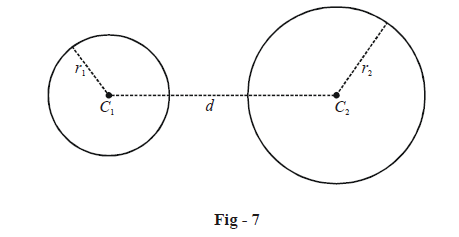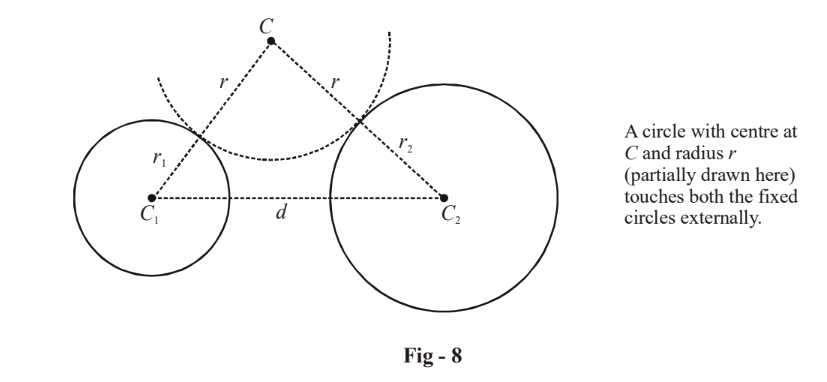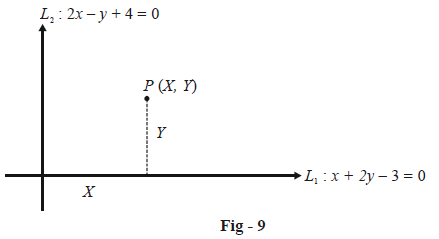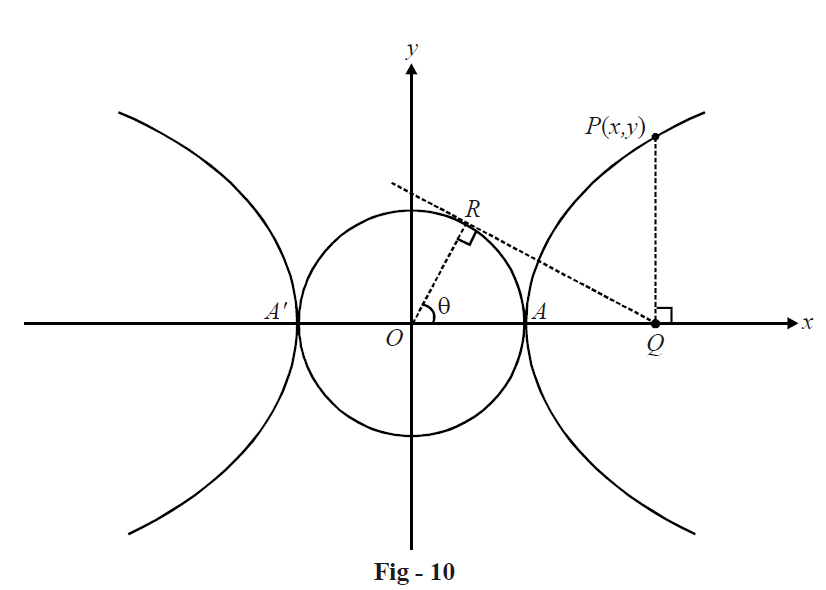# Basic Examples on Hyperbolas Set 2

Go back to  'Hyperbola'

Example - 4

What is the locus of the center of a circle which touches two given circles of radii $${r_1}$$ and $${r_2},$$ as shown below, externally ?Solution : Consider a circle of radius r and center at C, touching both the circles externally, as shown in the figure below :From the geometry of the figure, it is evident that

\begin{align}&\,\,\,\,\,\,\,\,\,\,\,\,\,\,\,\,\,\,\,\,\,\,\,\,\,\,\,\,\,\,\,\,C{C_1} = r + {r_1}\\\,\,\,\,\,\,\,\,\,\,\,\,\,\,\,\,\,\,\,\,\,\,\,\,\,\,\,\,\,\,\,\,&\qquad\qquad\quad\; C{C_2} = r + {r_2}\\ &\Rightarrow \left| {C{C_1} - C{C_2}} \right| = \left| {{r_1} - {r_2}} \right|\end{align}

This relation tells that the center C will always move in such a way so that the difference of its distances from two fixed points (the two centers C1 and C2) is always constant. Thus, the locus of the center C is a hyperbola with the two foci at C1 and C2.

The equation for the locus of C can easily be evaluated by first assuming a coordinate system with the origin at the mid-point of C1C2 and the x-axis along C1C2. Using standard symbols, we have

\begin{align}&\qquad\;2a = \left| {{r_1} - {r_2}} \right|\\&\qquad 2ae = d\\& \Rightarrow \quad e = \frac{d}{{\left| {{r_1} - {r_2}} \right|}}\end{align}

Since a and e are known, the equation for the hyperbola can now be written.

Example - 5

The equations of the transverse and conjugate axes of a hyperbola are $${L_1}:x + 2y - 3 = 0$$ and $${L_2}:2x - y + 4 = 0$$ respectively, and their respective lengths are $$\sqrt 2$$ and \begin{align}\frac{2}{{\sqrt 3 }}.\end{align} What is the equation of the hyperbola ?

Solution: We have,

\begin{align}&2a = \sqrt 2 \quad \Rightarrow \quad a = \frac{1}{{\sqrt 2 }}\\&2b = \frac{2}{{\sqrt 3 }} \quad \Rightarrow \quad b = \frac{1}{{\sqrt 3 }}\end{align}

We first find out the equation of the hyperbola in the $${L_1} - {L_2}$$ system ( in which the coordinates of any point can be represented by $$(X,\,\,Y)$$. This equation is simply

\begin{align}&\,\,\,\,\,\,\,\,\,\,\,\,\,\,\,\,\frac{{{X^2}}}{{{a^2}}} - \frac{{{Y^2}}}{{{b^2}}} = 1\\\\ &\Rightarrow \quad 2{X^2} - 3{Y^2} = 1\qquad\qquad\qquad\qquad ...\left( 1 \right)\end{align}

Now, what do X and Y represent? As we’ve already discussed in the previous chapter X and Y represent the perpendicular distances of the point P(X, Y) from $${L_2}$$ and $${L_1}$$ respectivelyThus, if in the original x - y system, P has the coordinates (x, y), we have,

$X = \frac{{\left| {2x - y + 4} \right|}}{{\sqrt 5 }};\,\,\,\,\,Y = \frac{{\left| {x + 2y - 3} \right|}}{{\sqrt 5 }}\,\,\,\,\,\,\,\,\,\,\,\,\,\,\,\,\,\,\,\,\,\,\,\,\,\,\,\,\,...\left( 2 \right)$

Using (2) in (1), the equation of the hyperbola is

$\frac{2}{5}{(2x - y + 4)^2} - \frac{3}{5}{(x + 2y - 3)^2} = 1$

which simplifies to

${x^2} - 4xy - 2{y^2} + 10x + 4y = 0$

Example - 6

For a variable parameter t, prove that the locus of the intersection point of the straight lines \begin{align}\frac{x}{a} - \frac{y}{b} = t\end{align} and \begin{align}\frac{x}{a} + \frac{y}{b} = \frac{1}{t}\end{align} is a hyperbola.

Solution: Let the point of intersection of the two lines be (h, k).

We have,

\begin{align}&\frac{h}{a} - \frac{k}{b} = t\\\\&\frac{h}{a} + \frac{k}{b} = \frac{1}{t}\end{align}

Multiplying the two relations (so that t gets eliminated), we obtain

$\frac{{{h^2}}}{{{a^2}}} - \frac{{{k^2}}}{{{b^2}}} = 1$

Thus, the locus of the intersection point is

$\frac{{{x^2}}}{{{a^2}}} - \frac{{{y^2}}}{{{b^2}}} = 1$

which is a hyperbola.

We will now consider how to represent a hyperbola in parametric form.

Consider the hyperbola \begin{align}\frac{{{x^2}}}{{{a^2}}} - \frac{{{y^2}}}{{{b^2}}} = 1.\end{align}On the transverse axis AA' as diameter, describe a circle. This circle will obviously touch both the segments of the hyperbola, as shown below. This circle is termed the auxiliary circle of the hyperbola:Let $$P(x,\,\,y)$$ be any point on the hyperbola. Drop a perpendicular PQ from P onto the transverse axis (the x-axis). From Q, draw the tangent QR to the auxiliary circle. In $$\Delta OQR,$$we have

\begin{align}&\;\;\;\;\,\,\,\,\,\,\,\,\,\frac{{OR}}{{OQ}} = \cos \theta \\ &\Rightarrow \quad OQ = OR\sec \theta = a\sec \theta \\ &\Rightarrow \quad x = a\sec \theta\end{align}

Thus, the x-coordinate of P in terms of $$\theta$$ is $$a \sec \theta .$$ Substituting $$x = a\sec \theta$$ in the equation of the hyperbola, we obtain $$y = b\tan \theta .$$ Therefore, we can conclude that any point \begin{align}P(x,\,\,y)\end {align} on the hyperbola \begin{align}\frac{{{x^2}}}{{{a^2}}} - \frac{{{y^2}}}{{{b^2}}} = 1\end{align} has the equivalent representation $$P(a\sec \theta ,\,b\tan \theta )$$:

$\boxed{P \equiv (x,\,y) \equiv (a\sec \theta ,\,\tan \theta )}$

This form is know as the parametric form of any point on the hyperbola, the parameter being  $$\theta$$  where $$0 \le \theta < 2\pi .$$ The point $$(a\sec \theta ,\,\,b\tan \theta )$$ is sometimes simply referred to as the point $$\theta .$$ (As in ellipses, $$\theta$$  is called the eccentric angle).

From Fig - 10, note that

$\frac{{PQ}}{{QR}} = \frac{{b\tan \theta }}{{a\tan \theta }} = \frac{b}{a} = {\rm{constant}}$

Thus, for any point P on the hyperbola, the ratio \begin{align}\frac{{PQ}}{{QR}}\end{align} is always fixed (where PQ and QR have been constructed as described above). In fact, the constancy of this ratio can itself be used to define a hyperbola (starting with the circle $${x^2} + {y^2} = {a^2},$$ we draw any tangent to it at a point R to intersect one of the circle’s diameter at Q. From Q, we construct a perpendicular PQ to this diameter, such that $$PQ:QR = \lambda$$ which is fixed. As R varies on the circle, P traces out hyperbola.

Download SOLVED Practice Questions of Basic Examples on Hyperbolas Set 2 for FREE
Hyperbolas
grade 11 | Questions Set 1
Hyperbolas
grade 11 | Answers Set 1
Hyperbolas
grade 11 | Questions Set 2
Hyperbolas
grade 11 | Answers Set 2
Learn from the best math teachers and top your exams

• Live one on one classroom and doubt clearing
• Practice worksheets in and after class for conceptual clarity
• Personalized curriculum to keep up with school